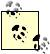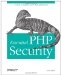# Section C.2. Using mcrypt

### C.2. Using mcrypt

The standard PHP extension for cryptography is mcrypt, and it supports a number of different cryptographic algorithms. To see which ones are supported on your platform, use the mcrypt_list_algorithms( ) function:

`     <?php     echo '<pre>' . print_r(mcrypt_list_algorithms(), TRUE) . '</pre>';     ?> `

Encrypting and decrypting data are achieved by using mcrypt_encrypt( ) and mcrypt_decrypt( ), respectively. Each of these functions accepts five arguments, the first of which is the algorithm to use:

`     <?php     mcrypt_encrypt(\$algorithm,                    \$key,                    \$cleartext,                    \$mode,                    \$iv);     mcrypt_decrypt(\$algorithm,                    \$key,                    \$ciphertext,                    \$mode,                    \$iv);     ?> `

The key (second argument) is extremely sensitive, so you want to be sure to keep this in a safe place. The technique described in Chapter 8 for protecting your database access credentials can be used to protect the key. A hardware key provides superior security, and this is the best choice for those who can afford it.

There are numerous modes that you can use, and you can use mcrypt_list_modes( ) to view a list of available modes:

`     <?php     echo '<pre>' . print_r(mcrypt_list_modes(), TRUE) . '</pre>';     ?> `

The fifth argument (\$iv) is the initialization vector, and it is created with the mcrypt_create_iv( ) function.

The following is an example class that offers basic methods for encrypting and decrypting:

`     class crypt     {       private \$algorithm;       private \$mode;       private \$random_source;       public \$cleartext;       public \$ciphertext;       public \$iv;       public function __construct(\$algorithm = MCRYPT_BLOWFISH,                                   \$mode = MCRYPT_MODE_CBC,                                   \$random_source = MCRYPT_DEV_URANDOM)       {         \$this->algorithm = \$algorithm;         \$this->mode = \$mode;         \$this->random_source = \$random_source;       }       public function generate_iv()       {         \$this->iv = mcrypt_create_iv(mcrypt_get_iv_size(\$this->algorithm,           \$this->mode), \$this->random_source);       }       public function encrypt()       {         \$this->ciphertext = mcrypt_encrypt(\$this->algorithm,           \$_SERVER['CRYPT_KEY'], \$this->cleartext, \$this->mode, \$this->iv);       }       public function decrypt()       {         \$this->cleartext = mcrypt_decrypt(\$this->algorithm,           \$_SERVER['CRYPT_KEY'], \$this->ciphertext, \$this->mode, \$this->iv);       }     }     ?> `

This class is referenced in other examples; the following example demonstrates its use:

`     <?php     \$crypt = new crypt();     \$crypt->cleartext = 'This is a string';     \$crypt->generate_iv();     \$crypt->encrypt();     \$ciphertext = base64_encode(\$crypt->ciphertext);     \$iv = base64_encode(\$crypt->iv);     unset(\$crypt);     /* Store \$ciphertext and \$iv (initialization vector). */     \$ciphertext = base64_decode(\$ciphertext);     \$iv = base64_decode(\$iv);     \$crypt = new crypt();     \$crypt->iv = \$iv;     \$crypt->ciphertext = \$ciphertext;     \$crypt->decrypt();     \$cleartext = \$crypt->cleartext;     ?> `This extension requires you to compile PHP with the with-mcrypt flag. See http://php.net/mcrypt for requirements and installation instructions.Essential PHP Security
ISBN: 059600656X
EAN: 2147483647
Year: 2005
Pages: 110

Similar book on Amazon

flylib.com © 2008-2017.
If you may any questions please contact us: flylib@qtcs.net